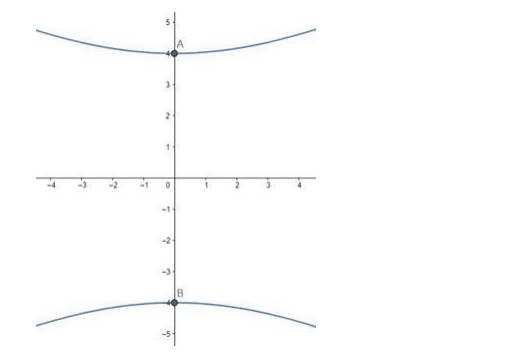# Find the value

Question:

Find the (i) lengths of the axes, (ii) coordinates of the vertices, (iii) coordinates of the foci, (iv) eccentricity and (v) length of the rectum of each of the following the hyperbola :

$\frac{y^{2}}{16}-\frac{x^{2}}{49}=1$

Solution:

Given Equation:

$\frac{y^{2}}{16}-\frac{x^{2}}{49}=1$

Comparing with the equation of hyperbola

$\frac{y^{2}}{a^{2}}-\frac{x^{2}}{b^{2}}=1$

we get,

a = 4 and b = 7(i) Length of Transverse axis $=2 \mathrm{a}=8$ units.

Length of Conjugate axis $=2 b=14$ units.

(ii) Coordinates of the vertices $=(0, \pm a)=(0, \pm 4)$

(iv) Here, eccentricity, $e=\sqrt{1+\frac{b^{2}}{a^{2}}}=\sqrt{1+\frac{49}{16}}=\sqrt{\frac{65}{16}}=\frac{\sqrt{65}}{4}$

(iii) Coordinates of the foci $=(0, \pm a e)=(0, \pm \sqrt{65})$

(v) Length of the rectum $=\frac{2 b^{2}}{a}=\frac{98}{4}=24.5$ units.